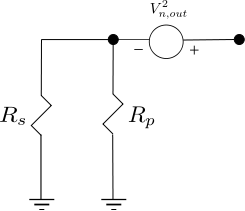- DSP log - http://www.dsplog.com -

Noise Figure of resistor network

Posted By Krishna Sankar On April 13, 2012 @ 3:17 am In Analog | 2 Comments

The post on thermal noise  described the noise produced by resistor  ohms over bandwidth  at temperature Kelvin. In this post, let us define the noise voltage at the input and output of a resistor network and further use it to define the Noise Figure of such a network.

From Section 2.3.2 of RF Microelectronics, Behzad Razavi, the noise figure is defined as

, where

is the input signal to noise ratio and

is the output signal to noise ratio.

Note : Some textbooks refer to this term as Noise Factor.

## Noise Figure of a resistor network

Consider a simple resistor circuit with  as the input voltage,  source resistance and  as the parallel resistance (shown below) :Figure : Resistor network (Reference : Figure 2.30(a) of  RF Microelectronics, Behzad Razavi)

The noise voltage due to the source resistance  is

.

The Signal to Noise Ratio at the input is,

.

The signal voltage at the output is,

The noise voltage at the output is,Figure : Equivalent resistor noise model for two parallel resistor (Reference : Figure 2.30(b) of  RF Microelectronics, Behzad Razavi )

.

The Signal to Noise Ratio at the output is,

.

Calculating,

.

The Noise Figure is,

Observations

a) Per the Maximum Power Transfer theorem  the source and load resistance should be equal i.e . However that condition does not coincide with the minimum noise figure. The source and load resistance and equal results in  (3dB in decibels)

b) Noise Figure is minimized by maximizing resistance  OR minimizing source resistance .

## Reference

RF Microelectronics, Behzad RazaviURL to article: http://www.dsplog.com/2012/04/13/noise-figure-resistor/

URLs in this post:

 thermal noise: http://www.dsplog.com/2012/03/25/thermal-noise-awgn/